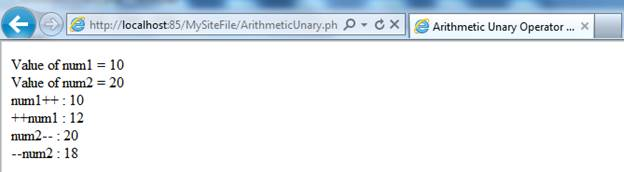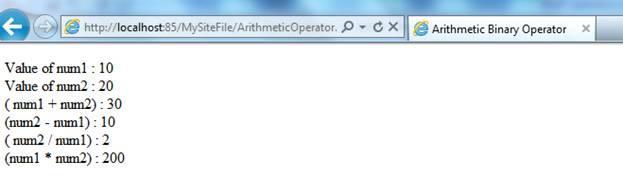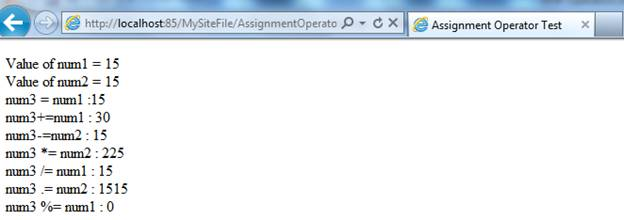Operators are used to operate on value. I.e. with the help of operator we can perform different types of actions on values such as increment, decrement, subtraction, division etc.

There are lots of operators in PHP such as Arithmetic operators, Assignment operators, Relational Operator and Logical Operators.

Let’s we have a brief idea about all these operators.

##### Arithmetic Operators:

Arithmetic operators are those operator which is used for numeric value calculation.

Let’s we have an example, which is showing a brief idea about Arithmetic operator.

Unary Operator: Unary operators appear before or after their operand and associate from right to left. I.e. Unary operators are those operators which operate on single operands. There are two important unary operator, ++ (increment operator) and - - (Decrement operator) used in php.

The unary operators are…

1.       ‘++’  (Increment Operator)

2.       ‘- -‘  (Decrement Operator)

Let’s we have an example, how to implement unary operator in php.

##### Example:
` <html>     <head>         <title>Arithmetic Unary Operator test</title>    </head><body>        <?php                       // Examples Arithmetic Operators           //  Declare variables              \$num1   = 10;            \$num2   = 20;        echo " Value of num1 = ".\$num1 ."</br>";       echo " Value of num2 = ".\$num2 ."</br>";              // post increment in num1       echo "num1++ : ".(\$num1++)."</br>";         // pre increment in num1       echo "++num1 : ".(++\$num1)."</br>";              // post decrement operator        echo "num2-- : ".(\$num2--)."</br>";         // pre decrement operator        echo "--num2 : ".(--\$num2)."</br>";         ?></body></html>`

##### Output:##### Binary Operator:

Binary operators are those operators which operate on two operands. Binary operator requires pair-//wise declaration. There are ma of binary operators used in php, some important are + (Addition), - (Subtraction), / (Division), *(Multiplication) etc.

Let’s we have an example, how to implement binary operator in php.

##### Example:
`<html>     <head>         <title>Arithmetic Unary Operator test</title>    </head><body>        <?php                       // Examples Arithmetic Operators           //  Declare variables              \$num1   = 10;            \$num2   = 20;        echo " Value of num1 = ".\$num1 ."</br>";       echo " Value of num2 = ".\$num2 ."</br>";             // post increment in num1      echo "num1++ : ".(\$num1++)."</br>";        // pre increment in num1       echo "++num1 : ".(++\$num1)."</br>";               // post decrement operator        echo "num2-- : ".(\$num2--)."</br>";          // pre decrement operator        echo "--num2 : ".(--\$num2)."</br>";         ?></body></html>`

##### Output:##### Assignment Operators:

Assignment operators are that operator which is used to assign one variable value into another one. There are lots of assignment operator used in php such as: =, +=, -=, *=, /=, .=, %= etc.

Let’s we have an example, which is showing a brief idea about Assignment operator.

##### Example:
`<html>     <head>         <title>Assignment Operator Test</title>    </head><body>        <?php                        //  Assignmentoperator   Example       // Declare variables         \$num1 = 15 ;       \$num2 =15;        echo "Value of num1 = ".\$num1."</br>";       echo "Value of num2 = ".\$num2."</br>";         // Equal (=) assignment operator       echo " num3 = num1   :".(\$num3 = \$num1)."</br>";              // '+=' Assignment operator        echo  "num3+=num1   : ".(\$num3 +=\$num1)."</br>";              // '-=' Assignment operator       echo "num3-=num2  : ".(\$num3 -=\$num2)."</br>";              //  '*=' Assignment operator       echo "num3 *= num2        : ".(\$num3 *=\$num2)."</br>";              // '/=' Assignment operator       echo "num3 /= num1     : ".(\$num3 /=\$num1)."</br>" ;         // '.=' Assignment operator here '.' (dot) is concatenation operator       echo " num3 .= num2    : ".(\$num3 .=\$num1)."</br>";         // '%=' Assignment operator       echo  "num3 %= num1   : ".(\$num3 %=\$num1)."</br>";         ?></body></html>`

##### Output:##### Relational Operator:

The relational operators compare two operands and determine the validity of a relationship. I.e.  Relational operator used for the comparison between two operands. There are lots of relational operator used in php such as: <, >, <=, >=, ==,!=, <> etc.

Let’s we have an example, How to implement relational operator in php.

Example (1):

`<html>      <head>        <title> Relational operator</title>      </head><body>        <?php              //  Declare variables                   \$val1 = 12;                   \$val2 = 24;                             if(\$val1 ==\$val2 )                {                      echo "Both are equal";                }               else                {                      echo "Both are not equal";                }                           ?></body>  </html>`

##### Output:Example (2):

`<html>      <head>        <title> Relational operator</title>      </head><body>        <?php              //  Declare variables                   \$val1 = 12;                   \$val2 = 24;                      if(\$val1 >\$val2 )                {                      echo "val1 is greater than val2";                 }              else if( \$val1 >= \$val2 )                 {                     echo "val1 is greater than or equal val2";                 }              else if( \$val1 <> \$val2)                 {                     echo "val1 is not equal to val2";                 }                   else                  {                     echo "above condition not apply";                  }                      ?></body>  </html>`

##### Logical Operator:

Logical operator operates on two boolean expressions and returns the boolean value. There are mainly three types of logical operator in php AND (&&) operator, OR (||) operator and NOT (!) operator.

Let’s we have an example, how to implement logical operators in php.

//Example:

`<html>      <head>        <title> Relational operator</title>      </head><body>        <?php              //  Declare variables                   \$x =12;                   \$y =24;    \$z = 25 ;                      if(\$x >\$y   &&  \$x <\$y )                {                     echo "This is AND (&&) operator block";                 }              if( \$x <\$y   || \$x >\$y)                 {                     echo "This is OR(||) operator block";                 }               if( ! \$x >\$y)                 {                     echo "This is NOT (!) operator block";                 }                             ?></body>  </html>`
##### Output:Modified On Sep-07-2019 12:12:34 AM